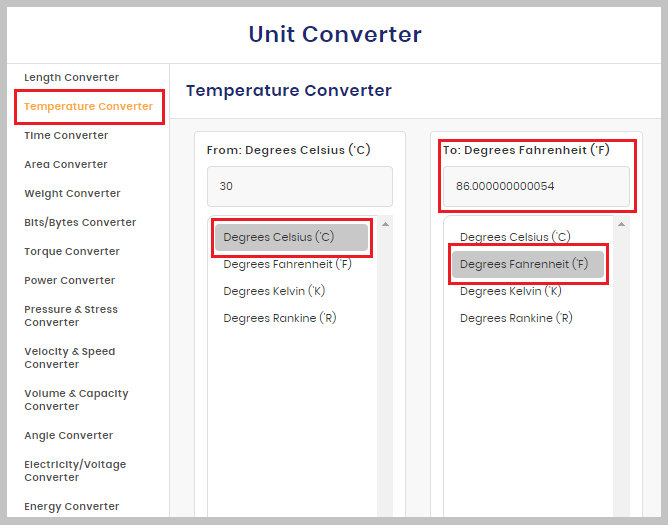# Temperature Conversion

Find out the differences between different units of temperatures by placing the quantity you wish to measure in the field located above the first column and select the unit from the next in which you want to transform the number to.

From: Meter(m)
To: Meter(m)

In order to convert between different temperature units, it would be necessary to use a temperature converter tool. This would afford you the benefit of transforming the basic units of temperature to any unit you require, anytime you need to.

Knowing the different units of temperature is essential, particularly when it is necessary to maneuver between the units and provide their equivalent in a different unit. You might be unable to calculate manually if you need to know the temperature right now.

Also, if you are wondering what’s the temperature in any unit, you can use this temperature calculator for easy Temperature Conversions.

Firstly, note that the basic units are Celsius (C) and Fahrenheit (F), and it is very common for people to need to Convert Celsius to Fahrenheit or convert Fahrenheit to Celsius. This is because some parts of the world, such as the United States and a number of Caribbean countries, make use of Fahrenheit as their unit of measurement. Other countries around the world are more familiar with Celsius as the unit for measuring temperature.

There is however the unit known as Kelvin (K), which is recognized by the International System of Units. The highest or boiling point of Celsius is measured at 100 and the lowest or freezing point at zero, while the highest and lowest point of Fahrenheit is at 212 and 32 respectively. But if you need to know at some point the temperature in Celsius, you can always calculate directly from Fahrenheit.

Although converting temperature units seems to be less complicated than other unit conversions, you get to save time and avoid errors when you simply use a Temperature Conversion tool. Within seconds, you can easily skip the whole manual Celsius to Fahrenheit formula with our temperature calculator.

At Search Engine Reports, our free online tool immediately helps you to know what’s the temperature in another unit. That is, you can know the temperature in Celsius or in Fahrenheit in an instant.

Our temp conversion has been made easy to use for all and sundry. Not only is it free and fast and super-accurate, it likewise requires zero installation stress because it is an online-based tool. It serves as a Celsius to Fahrenheit calculator, as well as a Fahrenheit to Celsius calculator. The troublesome nature of manually calculating units might become worrisome if you want to set a device to an exact temperature level. However, when you use our temperature converter tool, you easily convert F to C and can input the exactly accurate value.

To this temperature conversion calculator, you need not look too far. Simply follow these easy steps below:

- Visit https://searchenginereports.net/temperature-conversion to get started.

- On the page, ensure you have selected the temperature conversion tool, then select the temperature units you want to convert from, whether Celsius or Fahrenheit

- Input the value you want to calculate and then select the unit it should be converted into.

- The correct converted value immediately reflects below.It is thus obvious that temperature measurement units can be derived with a simple click when you use our great tool for your Celsius conversion. So perhaps you’re wondering, what’s the temperature right now, and you want the answer in a different unit of measurement. Simply calculate it instantly by exploring our online temperature calculator today.